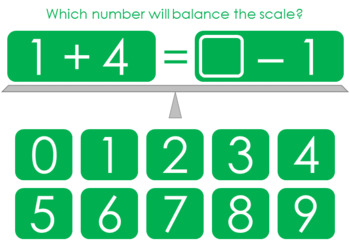# Balance the Equation Boom Cards Set 3eSubject
Resource Type
File Type

PDF

(597 KB|20 pages)
Standards
Also included in:
1. Balance the equation! Choose the answer that completes each equation to make the values on both sides of the scale equal. Great for practicing basic facts, introducing algebraic equations, and reinforcing number sense. Students will click on their response and receive immediate feedback, plus earn
\$5.00
\$4.00
Save \$1.00
2. Balance the equation! Choose the answer that completes each equation to make the values on both sides of the scale equal. Great for practicing basic facts, introducing algebraic equations, and reinforcing number sense. Students will click on their response and receive immediate feedback, plus earn p
\$15.25
\$12.20
Save \$3.05
• Product Description
• Standards
Balance the equation! Choose the answer that completes each equation to make the values on both sides of the scale equal. Great for practicing basic facts, introducing algebraic equations, and reinforcing number sense. Students will click on their response and receive immediate feedback, plus earn points! YOU know they’re learning; THEY think they’re playing!

What are Boom Cards?

Boom Cards live in the cloud. They can't be printed. They play on most modern browsers, Android, iPads, iPhones, and Kindle Fires. You open a Boom Learning account to play them (to protect the children). Create Fast Play pins to assign your Boom Cards to students.

Happy teaching!

:) Amanda
Fluently add and subtract within 20 using mental strategies. By end of Grade 2, know from memory all sums of two one-digit numbers.
Determine the unknown whole number in an addition or subtraction equation relating three whole numbers. For example, determine the unknown number that makes the equation true in each of the equations 8 + ? = 11, 5 = ▯ - 3, 6 + 6 = ▯.
Add and subtract within 20, demonstrating fluency for addition and subtraction within 10. Use strategies such as counting on; making ten (e.g., 8 + 6 = 8 + 2 + 4 = 10 + 4 = 14); decomposing a number leading to a ten (e.g., 13 - 4 = 13 - 3 - 1 = 10 - 1 = 9); using the relationship between addition and subtraction (e.g., knowing that 8 + 4 = 12, one knows 12 - 8 = 4); and creating equivalent but easier or known sums (e.g., adding 6 + 7 by creating the known equivalent 6 + 6 + 1 = 12 + 1 = 13).
Total Pages
20 pages
Included
Teaching Duration
N/A
Report this Resource to TpT
Reported resources will be reviewed by our team. Report this resource to let us know if this resource violates TpT’s content guidelines.